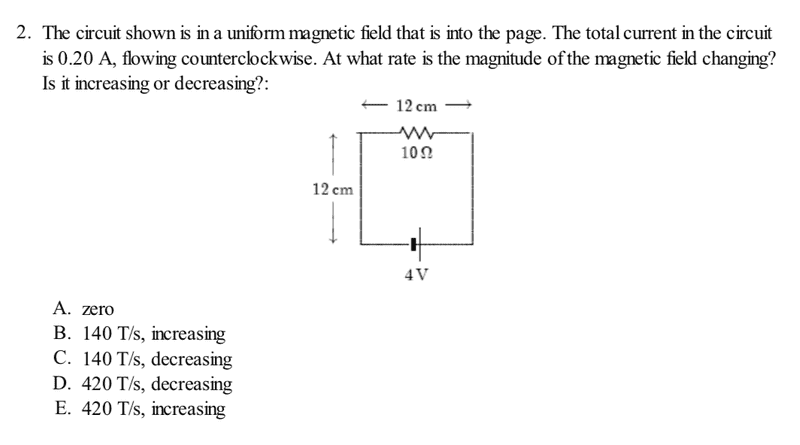# Rate of Change of Magnetic Field in Square wire loop

CDreyer23

## Homework Statement

The circuit shown is in a uniform magnetic field that is into the page. The total current in the circuit is 0.20 A, flowing counterclockwise. At what rate is the magnitude of the magnetic field changing? Is it increasing or decreasing?
Square wire loop with base and height of 12 cm
One 4 V battery and one 10 Ω resistor

## Homework Equations

(first ever post so sorry for the poor formatting)[/B]
dB = (μ0 * (I*ds)x(r))/(4πr2)
Bloop = (μ0*I)/(2r)

## The Attempt at a Solution

Given the direction of the current and using the right hand rule, the magnetic field produced by the current opposes the uniform one into the page, so it's magnitude is decreasing. However, the given equations don't fit with a square loop. I initially tried the second equation, but since r is changing it didn't work. I'm assuming the first equation is the one that's needed since we're looking for the change in magnetic field but I can't seem to find what ds should be.

Thanks for the help!

Homework Helper
Gold Member
There is no circuit shown. Can you post a figure?

CDreyer23
There is no circuit shown. Can you post a figure?
I'm not sure how to add a photo or a figure since there is no picture online it's on my professor's sample final exam. It is a square loop with side length of 12 cm. There is a 4V battery on the bottom and a 10 Ω resistor connected in series at the top. Current is moving counterclockwise, hope this is enough info to help

Homework Helper
Gold Member
Sometimes people post pictures that they take with their phones. If you do that, be sure everything is legible. It is not clear from your description why there should be a changing magnetic field.

CDreyer23